# A crib sheet for surfaces

[2018-02-02 Fri]

Every time I want to think about complex surfaces, I find I have forgotten what things like $$q(X)$$ and $$p_g(X)$$ mean and how they are related to things like $$c_1(X)$$ and $$c_2(X)$$. To save me carrying Barth-Peters-Van de Ven around with me, here are the basic definitions:

• $$q(X)$$ (irregularity) is the Hodge number $$h^{0,1}$$,
• $$p_g(X)$$ (geometric genus) is the Hodge number $$h^{0,2}$$,
• $$\chi(X)$$ (holomorphic Euler characteristic) is the alternating sum $$h^{0,2}-h^{0,1}+h^{0,0}$$,

so the Hodge diamond looks like this: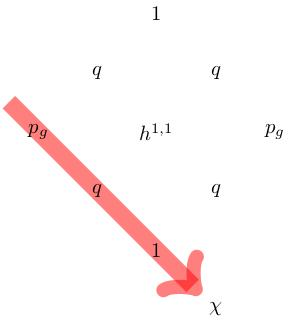## Characteristic classes and intersection form

A complex surface has characteristic numbers $$c_1^2$$, $$c_2$$ and $$p_1$$. The class $$c_2$$ equals the topological Euler characteristic of $$X$$. Since $$TX\otimes\mathbf{C}\cong TX\oplus (TX)^*$$ we get $p_1(X)=-c_2(TX\otimes\mathbf{C})=c_1^2(X)-2c_2(X).$ The Hirzebruch signature theorem tells us that the signature is given by $$\sigma=p_1/3=(2c_2-c_1^2)/3$$. We write $$b^+$$ and $$b^-$$ for the dimensions of the positive and negative eigenspaces of the intersection form.

One can relate the invariants $$q$$, $$p_g$$ and $$\chi$$ to the Chern numbers $$c_2$$, $$c_1^2$$ and the number $$b^+$$ as follows.

• By Hirzebruch-Riemann-Roch, we have $$\chi=\frac{1}{12}(c_1^2+c_2)$$ (which equals the Todd class of $$X$$). This is called Noether's formula.
• By the Hodge index theorem, the negative part of the intersection form is of dimension $$h^{1,1}-1$$, so we have $$b^+=2p_g+1$$,

## Examples

### $$\mathbf{CP}^2$$

The complex projective plane has $$q=p_g=0$$, $$\chi=1$$, $$b^+=1$$, $$c_1^2=9$$ and $$c_2=3$$. The Hodge diamond is: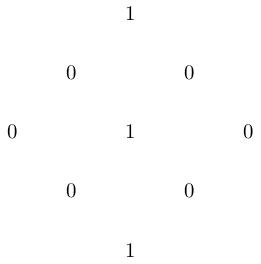### $$K3$$

A K3 surface has $$q=0$$, $$p_g=1$$, $$\chi=2$$, $$b^+=3$$, $$c_1^2=0$$ and $$c_2=24$$. The Hodge diamond is: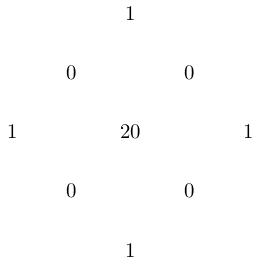### Hypersurface of degree $$d$$ in $$\mathbf{CP}^3$$.

Generalising the previous two examples ($$d=1,4$$), the hypersurface of degree $$d$$ in $$\mathbf{CP}^3$$ is simply-connected (by the Lefschetz hyperplane theorem) so $$q=0$$, and has $$c_1^2=d(d-4)^2$$, $$c_2=d(d^2-4d+6)$$ (see e.g. here for how to do this computation). This means $\chi=\frac{1}{6}d(d^2-6d+11)=p_g+1.$ For example, the Hodge diamond of the quintic surface ($$c_1^2=\chi=5$$, $$p_g=4$$, $$c_2=55$$) is therefore: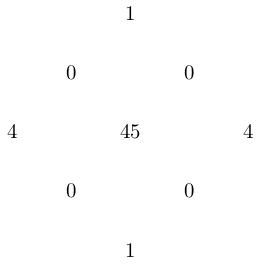## Minimal surfaces of general type

### Geography

The characteristic numbers of minimal surfaces of general type satisfy some further inequalities:

• Noether's inequality: $p_g\leq \frac{1}{2}c_1^2+2.$ When $$q=0$$, we have $$\frac{1}{12}(c_1^2+c_2)=\chi=p_g+1$$ and this inequality becomes $c_2\leq 5c_1^2+36.$
• The Bogomolov-Miyaoka-Yau inequality: $c_1^2\leq 3c_2.$
• $$c_1^2+c_2\equiv 0\mod 12$$ (by Noether's formula).
• $$c_1^2$$ and $$c_2$$ are positive.
If we plot the region bounded by these inequalities (assuming $$q=0$$) it looks like this: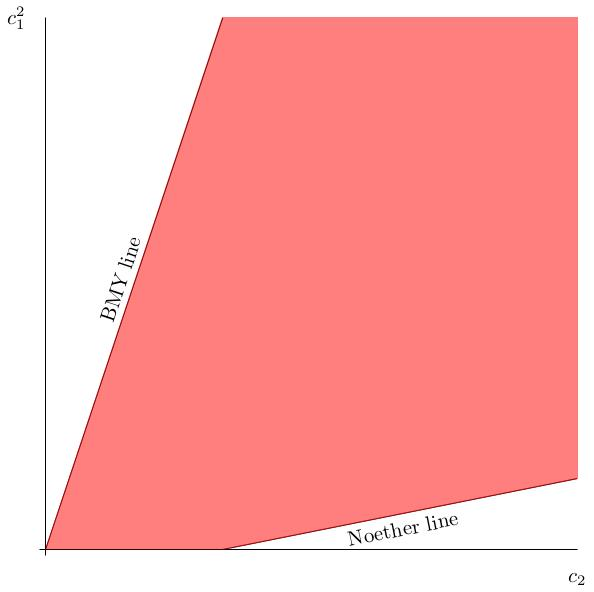### (Numerically) Godeaux surfaces ($$p_g=q=0$$, $$c_1^2=1$$)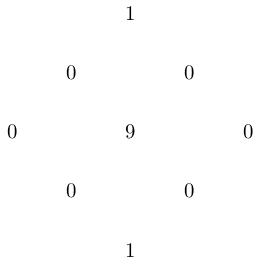These can have $$H_1(X;\mathbf{Z})=\mathbf{Z}/m$$ for $$m=1,2,3,4,5$$. The original Godeaux surface, with $$H_1=\mathbf{Z}/5$$, is obtained from the Fermat quintic $$x_0^5+\cdots+x_3^5=0$$ by quotienting by the free $$\mathbf{Z}/5$$-action where a fifth root of unity $$\mu$$ acts as $\mu(x_0,\ldots,x_3)=(x_0,\mu x_1,\mu^2 x_2,\mu^3 x_3).$ A simply-connected Godeaux surface was found by Barlow. For more on numerically Godeaux surfaces, see this paper by Reid.